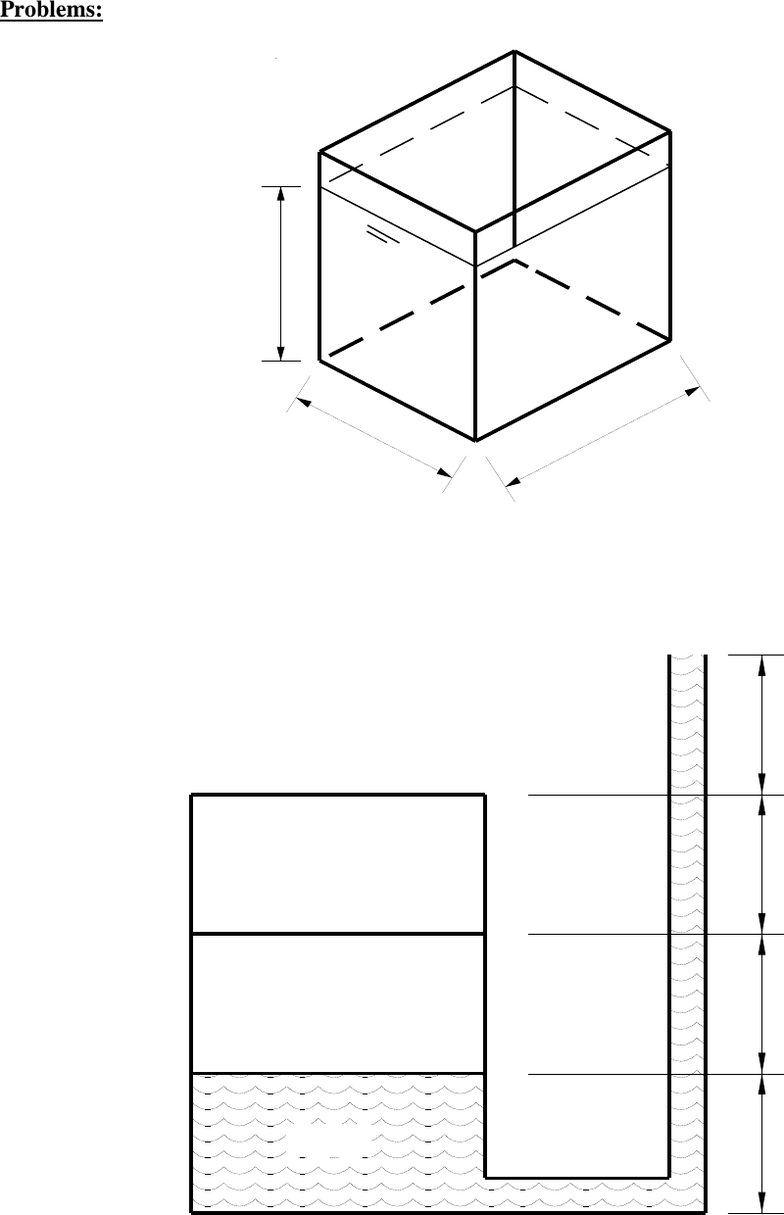# CIVE 3434 Lecture 3: Hydrostatic pressure 1(1)

56 views3 pagesProblems:
1.1 A tank shown below contains water, calculate the pressure at the bottom of tank.
Ans: 24525.0 N/m2
1.2 Convert intensity of pressure of 2 MPa into equivalent pressure head oil of relative density
0.8.
Ans: 254.842 m
1.3 Calculate the air pressure in the closed tank shown in Fig. below.
Ans.: 10791 N/m2
2.5 m
2.0 m
2.5 m
Air
Oil
sp. gr = 0.8
Water
0.5 m
0.5 m
0.5 m
0.5 m
Unlock document

This preview shows page 1 of the document.
Unlock all 3 pages and 3 million more documents.

## Document Summary

1. 1 a tank shown below contains water, calculate the pressure at the bottom of tank. m. 1. 2 convert intensity of pressure of 2 mpa into equivalent pressure head oil of relative density. 1. 3 calculate the air pressure in the closed tank shown in fig. below. 1. 4 determine the gauge pressure at the center of pipe b in kilopascals. Mercury (s = 13. 6) is the fluid in the manometer while oil (s = 0. 93) is the fluid flowing in the pipe. 1. 5 the differential mercury manometer, fig 1, measures the pressure of the water between point 1 and point 2. If the relative density of mercury is 13. 6 times that of water, what is the difference in pressure between point 1 and point 2 when h1 = 10 cm, z1 = 17 cm and z2. 1. 6 an inverted manometer is used to measure differential pressure in the water pipe.